# A reasonably speedy Python ray-tracer

Cyrille Rossant's small ray-tracer is an nice self-contained Python program (using NumPy) that renders this 400 × 300 image in about 15 seconds on a fast PC: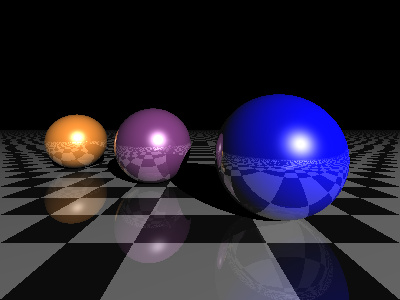You might conclude from this that a Python is an unsuitable language for a ray-tracer. But this version is slightly smaller and renders the same image in about 115 milliseconds. That's over 130 times faster than the original.

Both versions use NumPy, both run on a single core. The difference is how they organize their computation.

The original code looks a bit like this:

```for x in range(400):
for y in range(300):
pixel = raytrace(x, y)
write pixels to file
```

and the fast version looks more like this:

```x = <every pixel's x-coordinate from 0 to 400>
y = <every pixel's y-coordinate from 0 to 300>
pixels = raytrace(x, y)
write pixels to file
```

In the first version x and y are scalar values, and `raytrace()` runs 120,000 times. But in the second version x and y are NumPy arrays, each 120,000 values long, and `raytrace()` runs once. Because running Python code is quite slow, and NumPy's array operations are really fast, the speedup is huge.

NumPy lets you operate on arrays with no special syntax, so the actual definition of `raytrace()` doesn't need to be concerned much with the fact that it is operating on arrays instead of a scalars. For example the code to compute a sphere intersection looks similar to the scalar version:

```def intersect(self, O, D):
a = 1
b = 2 * D.dot(O - self.c)
c = abs(self.c) + abs(O) - 2 * self.c.dot(O) - (self.r * self.r)
disc = (b ** 2) - (4 * a * c)
sq = np.sqrt(np.maximum(0, disc))
h0 = (-b - sq) / 2
h1 = (-b + sq) / 2
h = np.where((h0 > 0) & (h0 < h1), h0, h1)

hit = (disc > 0) & (h > 0)
return np.where(hit, h, FARAWAY)
```

The only difference between this code and the scalar code is that it uses `np.where()` instead of `if` statements. This is because every expression is actually an array, so an `if` statement would not make the per-element selection that's required.

Starting with the generated rays, the code first runs `intersect()` on each of the objects in the scene, to find out their distance from the camera. Here lighter means further away:Taking the minimum of these distances gives the nearest 'hit' for every pixel: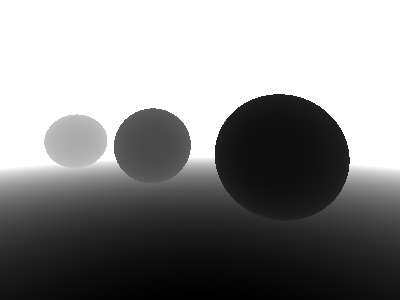Comparing each object's distance against the 'nearest' value gives four masks. A white pixel in the mask mean that that the object has 'won' the visibility competition.The next step is to run each object's shader function, to find out what its color is. This is done for every pixel on the screen, effectively rendering every object as a seperate image.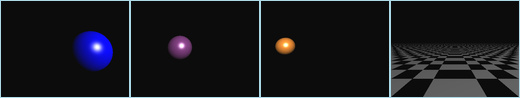Now `raytrace()` multiplies each color image with its mask, and sums the resulting four images. Because the masks determine which image is the 'nearest' for any particular pixel, the order doesn't matter. This gives this composite, a very basic first-bounce ray-trace: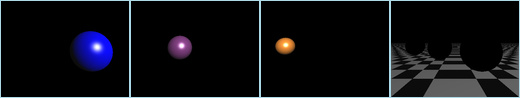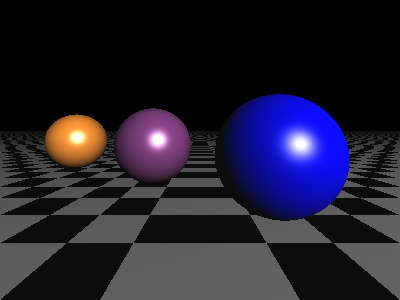From here on there are a few refinements, all involving a slightly fancier lighting function. Shadows are an important visual cue, and implementation is just a matter of testing each pixel to see if it can 'see' the light. If the pixel can see the light, it gets full brightness, otherwise it gets none: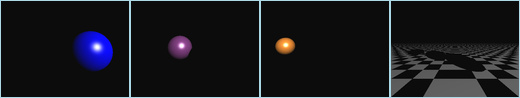Composited together, things look slightly more convincing: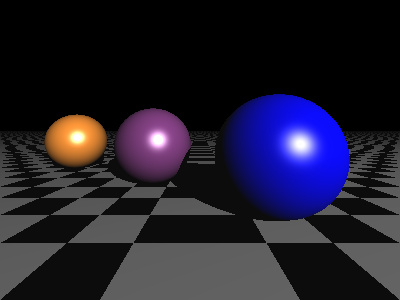The next step is the reflections, which is a recursive raytrace using the bounced ray from each pixel. This involves a lot of extra passes; the `raytrace()` function ends up being called 84 times with different combinations of secondary bounces: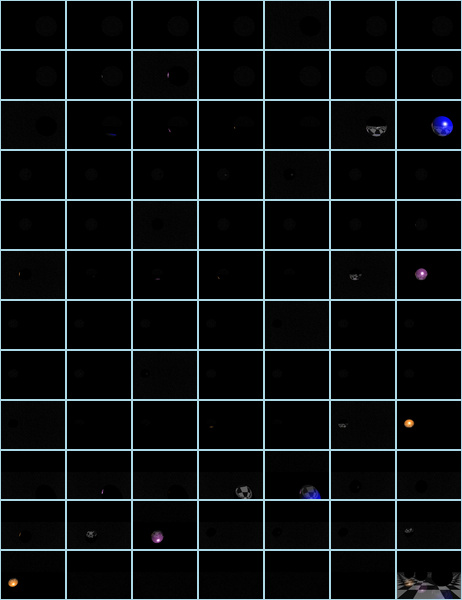The result matches the original quite well: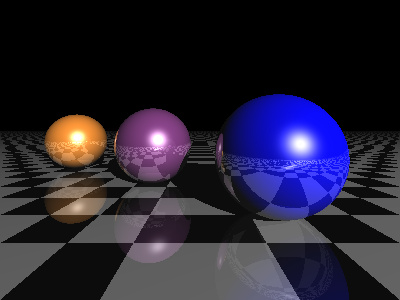Despite computing and compositing 84 ray-traced images, this version runs in about 4 seconds, one fourth of the time of the original. This version is rt2.py.

Speeding it up still further is a small optimization in `raytrace()`, exploiting the fact that the shader for any object only actually needs to run on that object's visible pixels. Using the masks computed for the intersection tests:and the Numpy `extract()` and `place()` functions, `raytrace()` squeezes out all the unused black pixels from each object's mask before it runs the object's shader. So if the object only covers 3000 pixels on the screen, then the NumPy arrays fed into the shader are only 3000 long, instead of 120,000. Because most objects don't actually cover much screen area, the speedup is huge, about 40X.

This version is rt3.py.